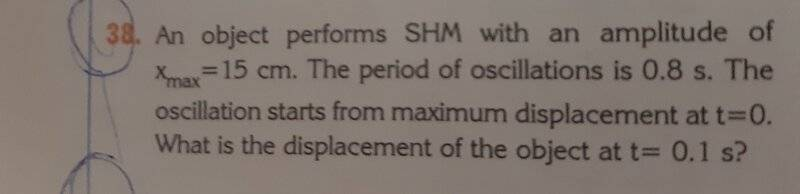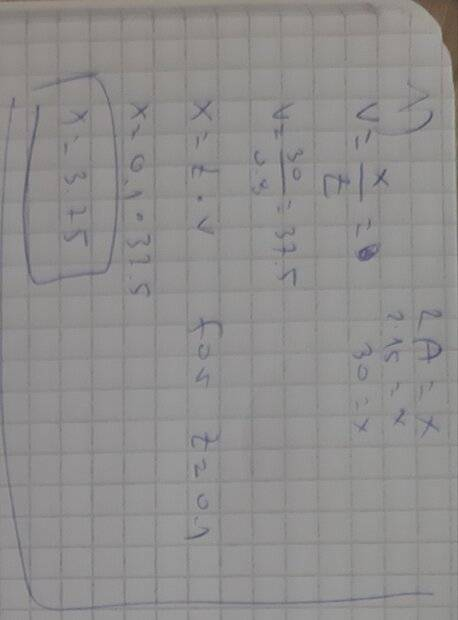# The 38th problem: I'm not getting the result in the book (SHM problem)

• Pawn
I didn't realize that pi was 3.14.I'm good now.Yes thank you so much for helping me .I didn't realize that pi was 3.14.I'm good now.

#### Pawn

Homework Statement
The 38 problem:The result on the book is 10.6cm i keep getting 3.75
Relevant Equations
X(t)=A×cos(w×t)Last edited:
Please show your work so that we can figure out where you went astray.

•topsquark
kuruman said:
Please show your work so that we can figure out where you went astray.

kuruman said:
Please show your work so that we can figure out where you went astray.Pawn said:

You are assuming that SHM is motion under zero acceleration. It is not. The velocity in SHM is not related to position and time by ##v=\frac{x}{t}##. What are the correct expressions for position and velocity in SHM? Your textbook should have those.

And yes, please learn how to write equations in LaTeX. Meanwhile, please do no post pictures sideways.

•MatinSAR
How can you get a displacement of 37.5 if the max amplitude is specified as 15?
(This is intended to inspire a sanity check. If you mentally draw this motion in your head, that may help you figure out where you're going wrong.)

•MatinSAR
kuruman said:
You are assuming that SHM is motion under zero acceleration. It is not. The velocity in SHM is not related to position and time by ##v=\frac{x}{t}##. What are the correct expressions for position and velocity in SHM? Your textbook should have those.

And yes, please learn how to write equations in LaTeX. Meanwhile, please do no post pictures sideways.
The only other equation i have is the one for the velocity in an instant of time v(t)=-vmax × sin(w × t).

W-omega
And i don't know what LaTeX is.

DaveC426913 said:
How can you get a displacement of 37.5 if the max amplitude is specified as 15?
(This is intended to inspire a sanity check. If you mentally draw this motion in your head, that may help you figure out where you're going wrong.)
3.75*

Pawn said:
3.75*
mm OK. Your notes say 3.75, though your problem statement says 37.5.
Not sure if you can still edit post #1.

Pawn said:
The only other equation i have is the one for the velocity in an instant of time v(t)=-vmax × sin(w × t).

W-omega
And i don't know what LaTeX is.
If your textbook really does not have an expression for the position as a function of time, look it up on the web.

To find how to use LaTeX to write and post equations, go here
https://www.physicsforums.com/help/latexhelp/
or click the link LaTeX Guide, lower left corner.

kuruman said:
If your textbook really does not have an expression for the position as a function of time, look it up on the web.

To find how to use LaTeX to write and post equations, go here
https://www.physicsforums.com/help/latexhelp/
or click the link LaTeX Guide, lower left corner.
Do you have any idea on how to solve it?

Knowing the period allows you to figure out ω. Knowing the position at t=0 allows you to figure out A. Then obtain x(t) for t=0.1s.

hutchphd said:
Knowing the period allows you to figure out ω. Knowing the position at t=0 allows you to figure out A. Then obtain x(t) for t=0.1s.
How ?

Look in your book for the relation between T and ω. Tell me what it is

hutchphd said:
Look in your book for the relation between T and ω. Tell me what it is
ω=2π/T

So in your equation for x $$x(t)=A\cos\frac {2\pi t} T$$ Now what is A?

A is 15cm. Right?

•hutchphd
so t/T is 1/8 for t=0.1s

hutchphd said:
So in your equation for x $$x(t)=A\cos\frac {2\pi t} T$$ Now what is A?
But if we substitute the values we don't get the result that is in the book.

Pawn said:
Do you have any idea on how to solve it?
I have lots of ideas how to solve it, but I will back off and let @hutchphd take over. You're in good hands.

•Pawn
cos (pi/4)= ? ( that's 45deg)

hutchphd said:
cos (pi/4)= ?
Do we substitute pi with the value 3.14

You prefer 2pi/8 ? see #18

hutchphd said:
You prefer 2pi/8 ? see #18
No sorry i didnt realize it

hutchphd said:
cos (pi/4)= ? ( that's 45deg)
0.70

So are you good? I got to go right now but let me know

hutchphd said:
So are you good? I got to go right now but let me know
Yes thank you so much for helping me .

•berkeman and hutchphd